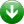Sunday, June 16, 2019
Home Search

matlab - search results

If you're not happy with the results, please do another search

Real-Time Face Detection Using MATLAB

Object detection and tracking are important in many computer vision applications, including activity recognition, automotive safety and surveillance. Presented here is an face detection using...

Going Beyond the Intelligence of Artificial Intelligence at MATLAB Expo’19

The latest Artificial Intelligence (AI) forecast by McKinsey says that AI will create \$13 trillion in value by 2020. This is the pace at...

Image Processing Using MATLAB: Basic Operations (Part 1 of 4)

In this series of four articles, fundamentals, as well as advanced topics of image processing using MATLAB, are discussed. The articles cover basic to...

Wireless Control of Robotic Car Through MATLAB GUI

This project presents the implementation of a MATLAB graphical user interface (GUI)-based approach to wirelessly control the movement of a robotic car. Commands to...

Basic Image Processing Using MATLAB

In this article, the author describes basic image processing using MATLAB software. MATLAB is a high-performance language for technical computing with powerful commands and syntax....

Top 25 MATLAB Project Ideas

Millions of engineers and scientists worldwide use MATLAB to analyze and design the systems and products transforming our world. Such huge usage leads to...

Logging Sensor Data in MS EXCEL through MATLAB GUI

The measurement and analysis of process parameters such as temperature, flow, conductivity, speed, viscosity and stress play a crucial role in providing information about...

What Is the Difference Between LabVIEW and MATLAB?

LabVIEW, or Laboratory Virtual Instrument Engineering Workbench, is a system design platform and development environment for graphical language programming. It is a visual programming...

Light Animations Using Arduino and MATLAB

Light animations are visually appealing and hence widely used for advertising purposes. In this project, we present a MATLAB-based graphical user interface (GUI) approach...

R-, G-, B- Histogram Extraction of a True Colour Image (JPEG) Using MATLAB

The images in JPEG format are true colour images with 24-bit colour resolution. A 24-bit colour image is the combination of R-, G- and...

950,990Fans
564Followers
20,751Subscribers